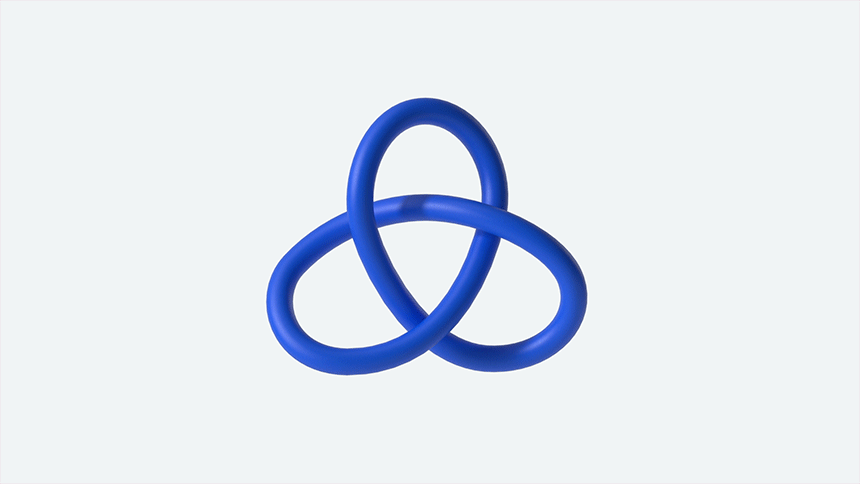# AI Is Discovering New Patterns In Pure Mathematics – Some Of Them Never Seen BeforeUsing artificial intelligence (AI) and machine learning, scientists have created a new mathematical theorem. This discovery is the first to show that artificial intelligence can help with pure mathematics.

While mathematicians have been using computers to find patterns for decades, the increased capability of machine learning means that these networks can now sift through massive amounts of data and find patterns that have never been seen before.

To research knot theory and representation theory, mathematicians from the universities of Oxford and Sydney collaborated with DeepMind, a Google-owned firm based in London.

Both of these are considered pure mathematics, and their growth is strongly reliant on human intuition. According to the researchers in a recent article, DeepMind was able to detect patterns and links in the two complex mathematical fields.

The team showed AI progressing a proof for Kazhdan-Lusztig polynomials, a 40-year-old math problem involving the symmetry of higher-dimensional algebra.

The study also showed how a supervised learning model, a machine learning technique, was able to spot a previously unknown relationship between two types of mathematical knots, leading to an altogether new theorem.A knot is one of the fundamental objects in low-dimensional topology. It is a twisted loop embedded in 3 dimensional space. (Credit: DeepMind)

András Juhász, a mathematician at Oxford said that “we have demonstrated that, when guided by mathematical intuition, machine learning provides a powerful framework that can uncover interesting and provable conjectures in areas where a large amount of data is available, or where the objects are too large to study with classical methods,”.

The study found that high-speed, ultra-reliable, large-scale data processing can be used as a supplement to mathematicians’ inherent intuition. That can make a big impact when you’re dealing with long, intricate formulae.

The researchers believe that their study will lead to many more collaborations between academics in the domains of mathematics and artificial intelligence, allowing for hitherto uncovered discoveries.

Previous article

Next article